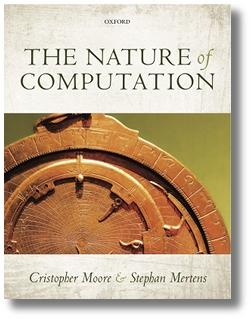Complexity Explorer Santa Fe Institute

This course is no longer in session.Computation in Complex Systems (summer 2021)

• Course Introduction
• Two Kinds of Paths
• Polynomials vs. Exponentials
• Divide and Conquer
• Big O and All that
• When the details don't matter
• Quiz 1 (self-assessment)
• Exam
• Divide and Conquer Redux
• Dynamic Programming
• Greedy Algorithms
• Reductions and Translations
• Lessons So Far
• The Best of All Possible Algorithms
• Complexity Wrap-Up
• Quiz 2 (self-assessment)
• Exam
• Finding versus Checking
• Circuits and Formulas
• More NP-complete Problems
• P versus NP Problem
• Existence and Nonexistence
• Above and Beyond
• Exam
• Real World Problems
• Phase Transitions
• Random Problems
• Solvability Threshold
• Modeling Differential Equations
• Landscapes, Clustering, Freezing, and Hardness
• Exam
• Building Blocks: Recursive Functions
• Building Blocks: Partial Recursive Functions
• λ Calculus
• Turing Machines
• The Halting Problem
• The Grand Unified Theory of Computation
• The Analytical Engine
• Cellular Automata
• Tile-Based Computation
• Dynamical Systems
• Quiz 5 (self-assessment)
• Exam
• More from Cris Moore
• Other ComplexityExplorer resources

6.1 More from Cris Moore » The Nature of Computation

If you enjoyed the introduction to computational theory in this course, you can find more detail and discussion in The Nature of Computation. The book's website has a few sample chapters. There is a solutions manual for those interested in using the book for classroom instruction.